# Independent Samples tTest or 2 Sample tTest Advanced

• Slides: 37Independent Samples t-Test (or 2 -Sample t-Test) Advanced Research Methods in Psychology - lecture Matthew Rockloff 1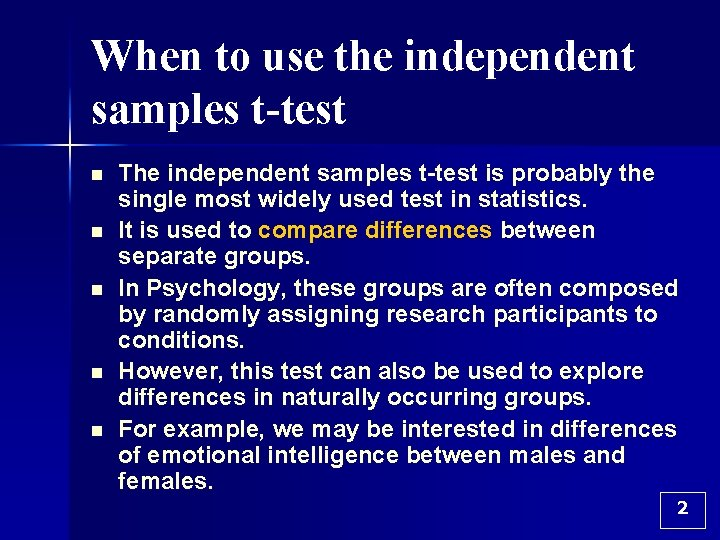When to use the independent samples t-test n n n The independent samples t-test is probably the single most widely used test in statistics. It is used to compare differences between separate groups. In Psychology, these groups are often composed by randomly assigning research participants to conditions. However, this test can also be used to explore differences in naturally occurring groups. For example, we may be interested in differences of emotional intelligence between males and females. 2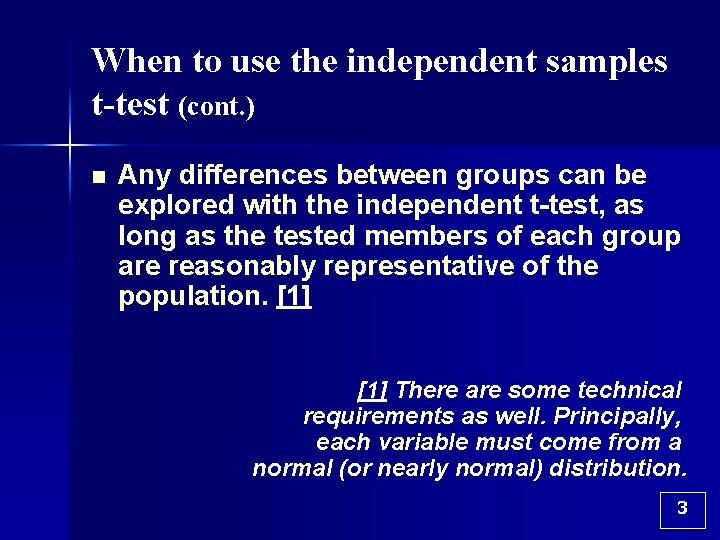When to use the independent samples t-test (cont. ) n Any differences between groups can be explored with the independent t-test, as long as the tested members of each group are reasonably representative of the population.  There are some technical requirements as well. Principally, each variable must come from a normal (or nearly normal) distribution. 3Example 3. 1 n n n Suppose we put people on 2 diets: the pizza diet and the beer diet. Participants are randomly assigned to either 1 -week of eating exclusively pizza or 1 -week of exclusively drinking beer. Of course, this would be unethical, because pizza and beer should always be consumed together, but this is just an example. 4Example 3. 1 (cont. ) n At the end of the week, we measure weight gain by each participant. n Which diet causes more weight gain? n In other words, the null hypothesis is: Ho: wt. gain pizza diet =wt. gain beer diet. 5Example 3. 1 (cont. ) n n Why? The null hypothesis is the opposite of what we hope to find. In this case, our research hypothesis is that there ARE differences between the 2 diets. Therefore, our null hypothesis is that there are NO differences between these 2 diets. 6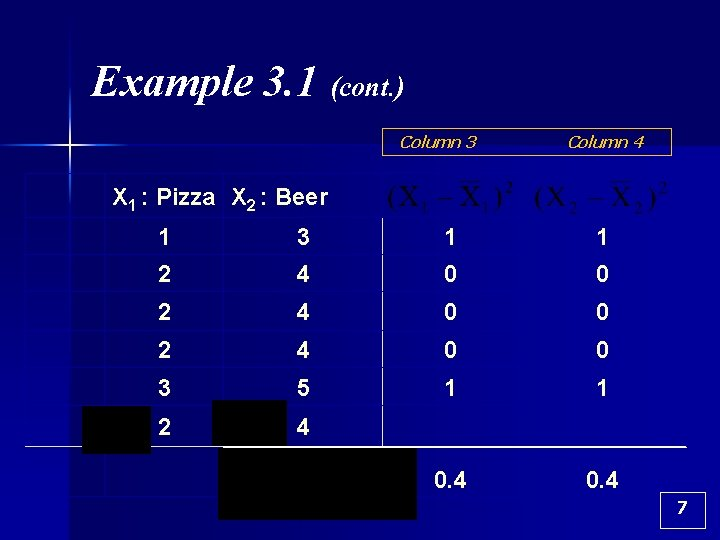Example 3. 1 (cont. ) Column 3 Column 4 X 1 : Pizza X 2 : Beer 1 3 1 1 2 4 0 0 3 5 1 1 2 4 0. 4 7Example 3. 1 (cont. ) n n n The first step in calculating the independent samples t-test is to calculate the variance and mean in each condition. In the previous example, there a total of 10 people, with 5 in each condition. Since there are different people in each condition, these “samples” are “independent” of one another; giving rise to the name of the test. 8Example 3. 1 (cont. ) n n n The variances and means are calculated separately for each condition (Pizza and Beer). A streamlined calculation of the variance for each condition was illustrated previously. (See Slide 7. ) In short, we take each observed weight gain for the pizza condition, subtract it from the mean gain of the pizza dieters ( 2) and square the result (see column 3). 9Example 3. 1 (cont. ) n Next, add up column 3 and divide by the number of participants in that condition (n 1 = 5) to get the sample variance, n The same calculations are repeated for the “beer” condition. 10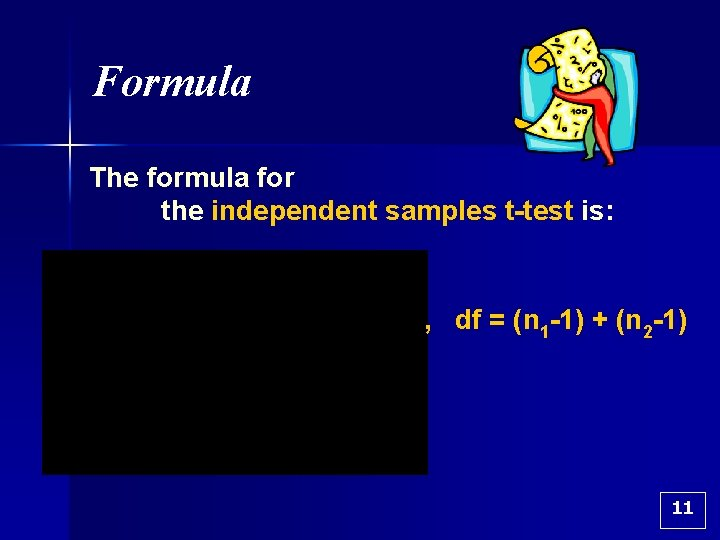Formula The formula for the independent samples t-test is: , df = (n 1 -1) + (n 2 -1) 11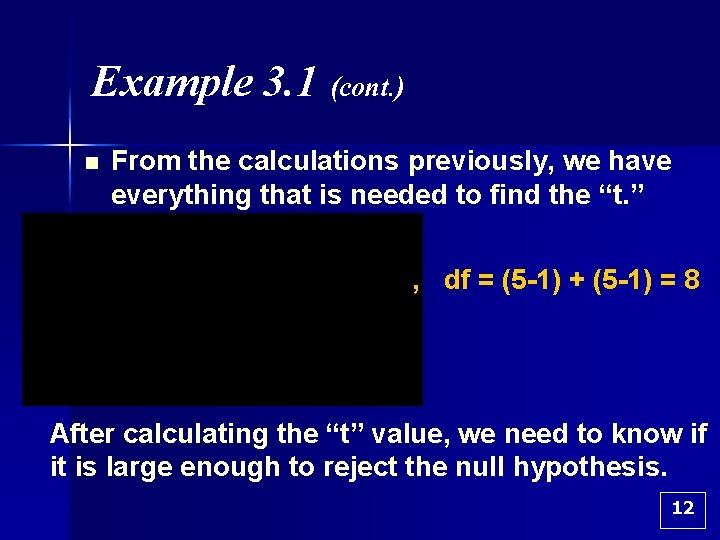Example 3. 1 (cont. ) n From the calculations previously, we have everything that is needed to find the “t. ” , df = (5 -1) + (5 -1) = 8 After calculating the “t” value, we need to know if it is large enough to reject the null hypothesis. 12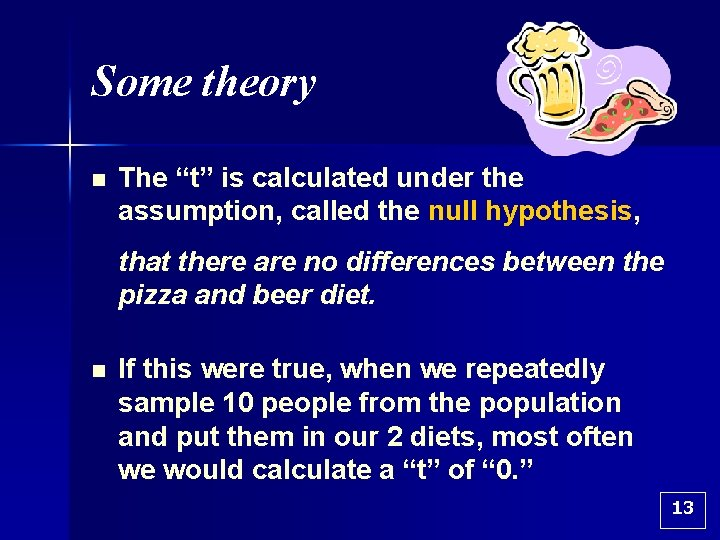Some theory n The “t” is calculated under the assumption, called the null hypothesis, that there are no differences between the pizza and beer diet. n If this were true, when we repeatedly sample 10 people from the population and put them in our 2 diets, most often we would calculate a “t” of “ 0. ” 13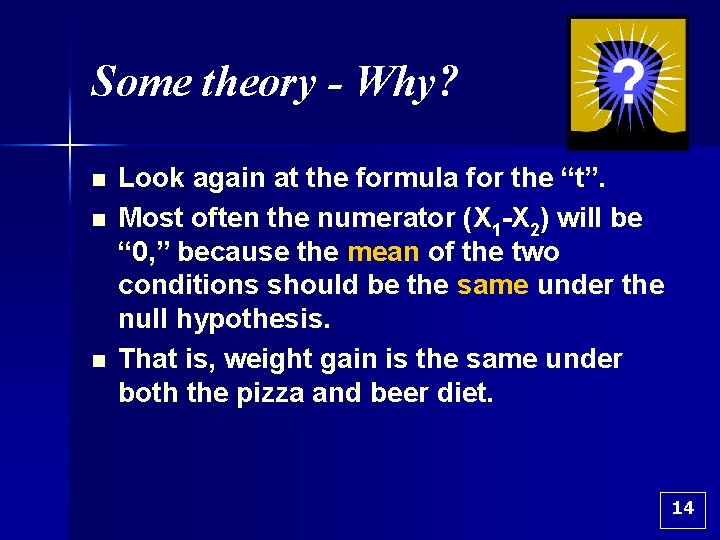Some theory - Why? n n n Look again at the formula for the “t”. Most often the numerator (X 1 -X 2) will be “ 0, ” because the mean of the two conditions should be the same under the null hypothesis. That is, weight gain is the same under both the pizza and beer diet. 14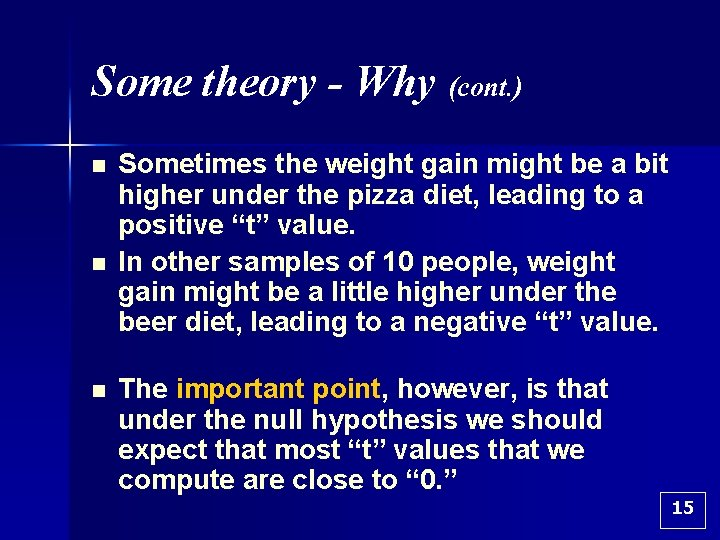Some theory - Why (cont. ) n n n Sometimes the weight gain might be a bit higher under the pizza diet, leading to a positive “t” value. In other samples of 10 people, weight gain might be a little higher under the beer diet, leading to a negative “t” value. The important point, however, is that under the null hypothesis we should expect that most “t” values that we compute are close to “ 0. ” 15Some theory (cont. ) n n Our computed t-value is not “ 0, ” but it is in fact negative (t(8) = -4. 47). Although the t-value is negative, this should not bother us. Remember that the t-value is only - 4. 47 because we named the pizza diet X 1 and the beer diet X 2. – This is, of course, completely arbitrary. If we had reversed our order of calculation, with the pizza diet as X 2 and the beer diet as X 1, then our calculated t-value would be positive 4. 47. 16Example 3. 1 (again) Calculations n n The calculated t-value is 4. 47 (notice, I’ve eliminated the unnecessary “-“ sign), and the degrees of freedom are 8. In the research question we did not specify which diet should cause more weight gain, therefore this t-test is a socalled “ 2 -tailed t. ” 17Example 3. 1 (again) Calculations n n In the last step, we need to find the critical value for a 2 -tailed “t” with 8 degrees of freedom. This is available from tables that are in the back of any Statistics textbook. Look in the back for “Critical Values of the t-distribution, ” or something similar. The value you should find is: C. V. t(8), 2 -tailed = 2. 31. 18Example 3. 1 (cont. ) n n The calculated t-value of 4. 47 is larger in magnitude than the C. V. of 2. 31, therefore we can reject the null hypothesis. Even for a results section of journal article, this language is a bit too formal and general. It is more important to state the research result, namely: Participants on the Beer diet (M = 4. 00) gained significantly more weight than those on the Pizza diet (M = 2. 00), t(8) = 4. 47, p <. 05 (two-tailed). 19Example 3. 1 (concluding comment) Repeat from previous slide: Participants on the Beer diet (M = 4. 00) gained significantly more weight than those on the Pizza diet (M = 2. 00), t(8) = 4. 47, p <. 05 (two-tailed). n Making this conclusion requires inspection of the mean scores for each condition (Pizza and Beer). 20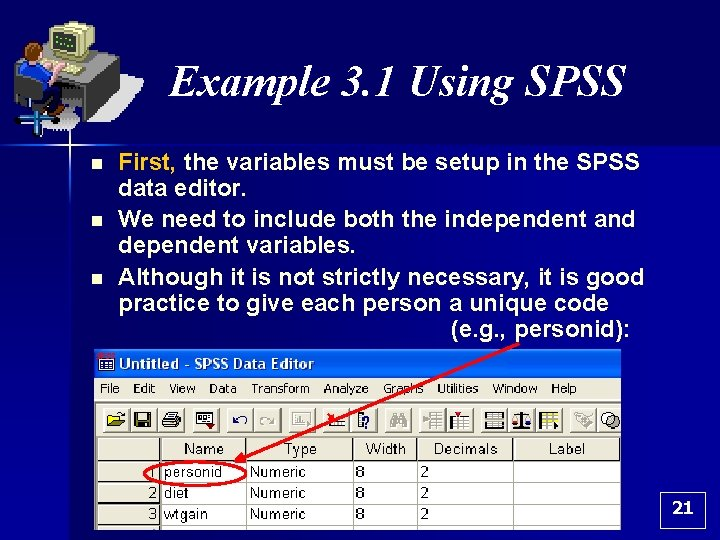Example 3. 1 Using SPSS n n n First, the variables must be setup in the SPSS data editor. We need to include both the independent and dependent variables. Although it is not strictly necessary, it is good practice to give each person a unique code (e. g. , personid): 21Example 3. 1 Using SPSS (cont. ) n In the previous example: – Dependent Variable = wtgain (or weight gain) – Independent Variable = diet n Why? n The independent variable (diet) causes changes in the dependent variable (weight gain). 22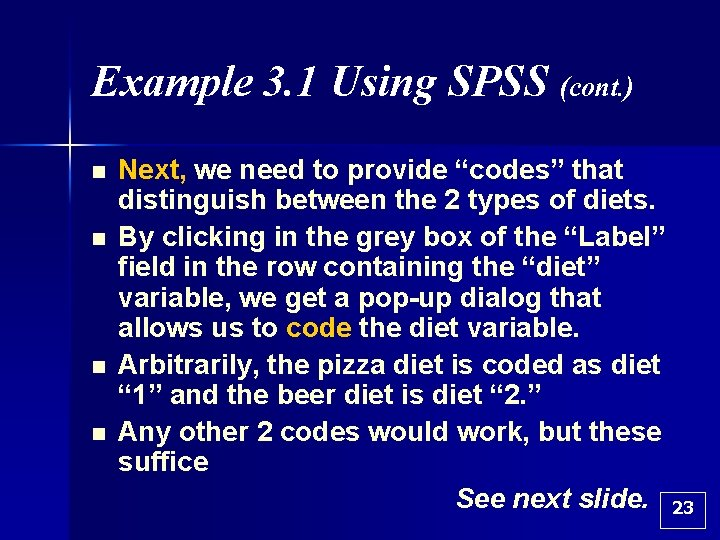Example 3. 1 Using SPSS (cont. ) n n Next, we need to provide “codes” that distinguish between the 2 types of diets. By clicking in the grey box of the “Label” field in the row containing the “diet” variable, we get a pop-up dialog that allows us to code the diet variable. Arbitrarily, the pizza diet is coded as diet “ 1” and the beer diet is diet “ 2. ” Any other 2 codes would work, but these suffice See next slide. 23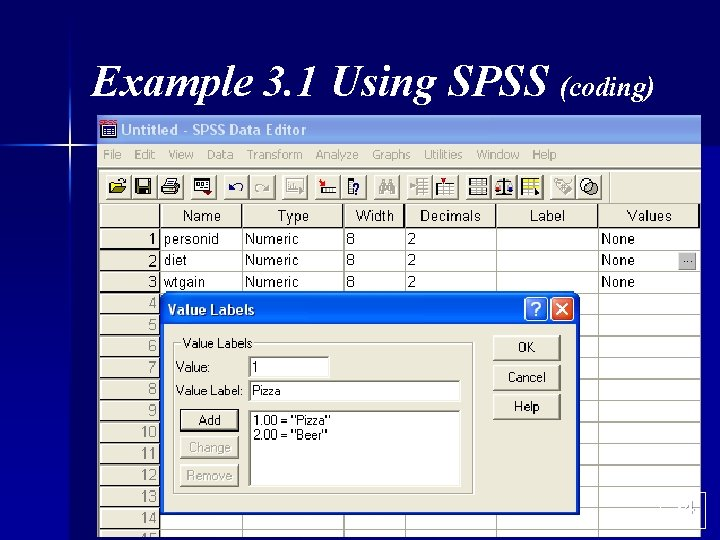Example 3. 1 Using SPSS (coding) 24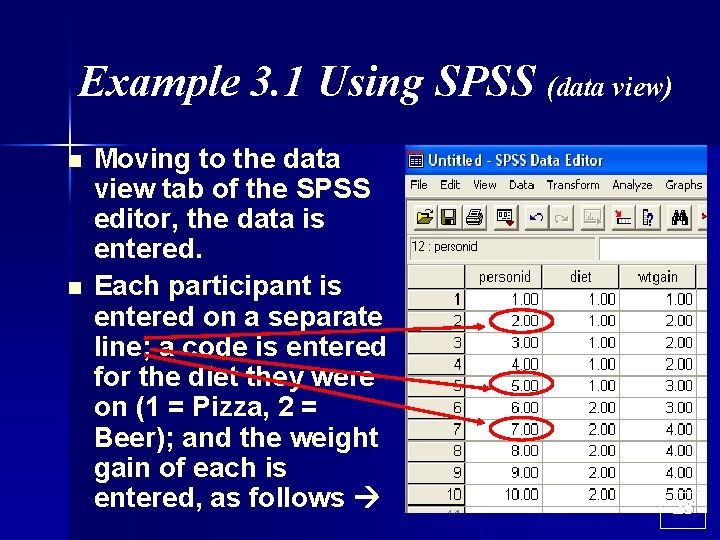Example 3. 1 Using SPSS (data view) n n Moving to the data view tab of the SPSS editor, the data is entered. Each participant is entered on a separate line; a code is entered for the diet they were on (1 = Pizza, 2 = Beer); and the weight gain of each is entered, as follows 25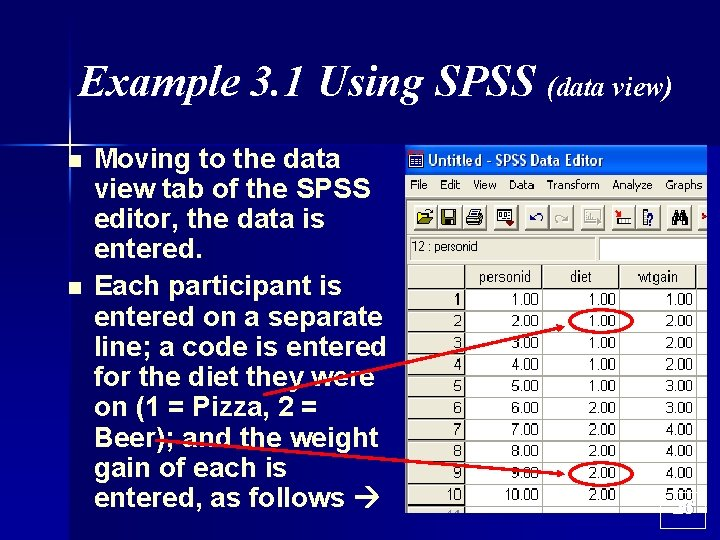Example 3. 1 Using SPSS (data view) n n Moving to the data view tab of the SPSS editor, the data is entered. Each participant is entered on a separate line; a code is entered for the diet they were on (1 = Pizza, 2 = Beer); and the weight gain of each is entered, as follows 26Example 3. 1 Using SPSS (data view) n n Moving to the data view tab of the SPSS editor, the data is entered. Each participant is entered on a separate line; a code is entered for the diet they were on (1 = Pizza, 2 = Beer); and the weight gain of each is entered, as follows 27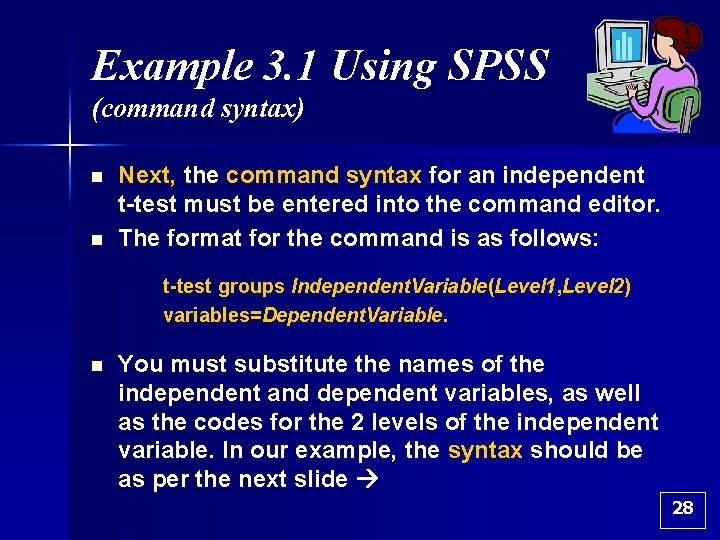Example 3. 1 Using SPSS (command syntax) n n Next, the command syntax for an independent t-test must be entered into the command editor. The format for the command is as follows: t-test groups Independent. Variable(Level 1, Level 2) variables=Dependent. Variable. n You must substitute the names of the independent and dependent variables, as well as the codes for the 2 levels of the independent variable. In our example, the syntax should be as per the next slide 28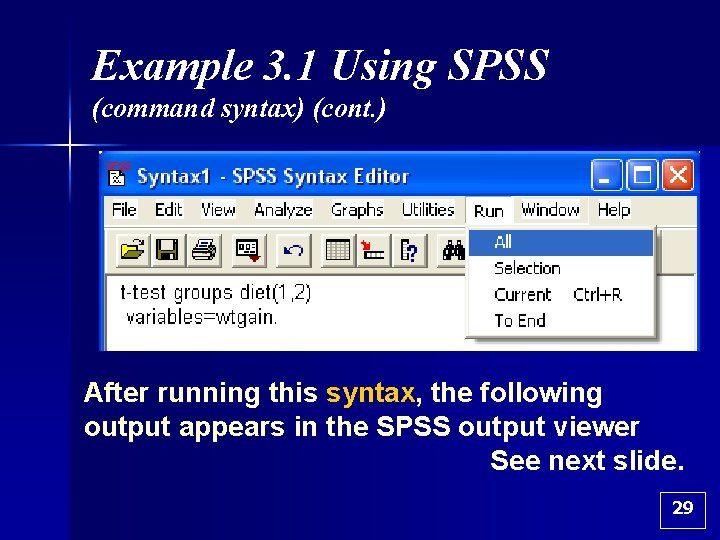Example 3. 1 Using SPSS (command syntax) (cont. ) After running this syntax, the following output appears in the SPSS output viewer See next slide. 29Example 3. 1: SPSS Output viewer Independent Samples Test 30Example 3. 1 Using SPSS (cont. ) n n n SPSS gives the means for each of the conditions (Pizza Mean = 2 and Beer Mean = 4). In addition, SPSS provides a t-value of 4. 47 with 8 degrees of freedom. These are the same figures that were computed “by hand” previously. However, SPSS does not provide a critical value. Instead, an exact probability is provided (p =. 002). 31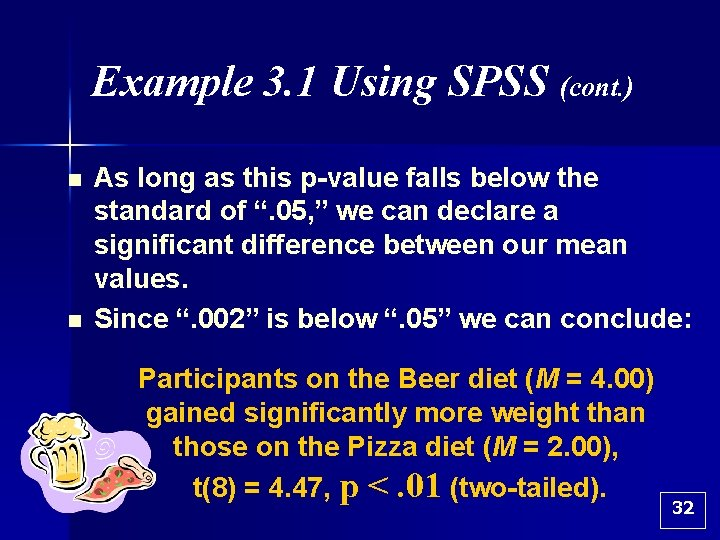Example 3. 1 Using SPSS (cont. ) n n As long as this p-value falls below the standard of “. 05, ” we can declare a significant difference between our mean values. Since “. 002” is below “. 05” we can conclude: Participants on the Beer diet (M = 4. 00) gained significantly more weight than those on the Pizza diet (M = 2. 00), t(8) = 4. 47, p <. 01 (two-tailed). 32Example 3. 1 Using SPSS (cont. ) Repeat from previous slide: Participants on the Beer diet (M = 4. 00) gained significantly more weight than those on the Pizza diet (M = 2. 00), t(8) = 4. 47, p <. 01 (two-tailed). n n In APA style we normally only display significance to 2 significant digits. Therefore, the probability is displayed as “p<. 01, ” which is the smallest probability within this range of accuracy. 33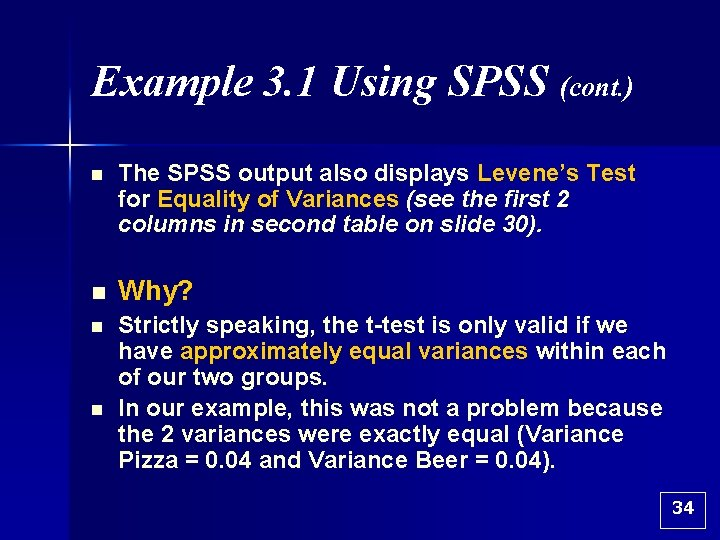Example 3. 1 Using SPSS (cont. ) n The SPSS output also displays Levene’s Test for Equality of Variances (see the first 2 columns in second table on slide 30). n Why? n Strictly speaking, the t-test is only valid if we have approximately equal variances within each of our two groups. In our example, this was not a problem because the 2 variances were exactly equal (Variance Pizza = 0. 04 and Variance Beer = 0. 04). n 34Example 3. 1 Using SPSS (cont. ) n n However, if this test is significant, meaning that the p-value given is less than “. 05, ” then we should choose the bottom line when interpreting our results. This bottom line makes slight adjustments to the t-test to account for problems when there are not equal variances in both conditions. 35Example 3. 1 Using SPSS (cont. ) n n The practical importance of this distinction is small. Even if variances are not equal between conditions, the hand calculations we have shown will most often lead to the correct conclusion anyway, and use of the “top line” is almost always appropriate. 36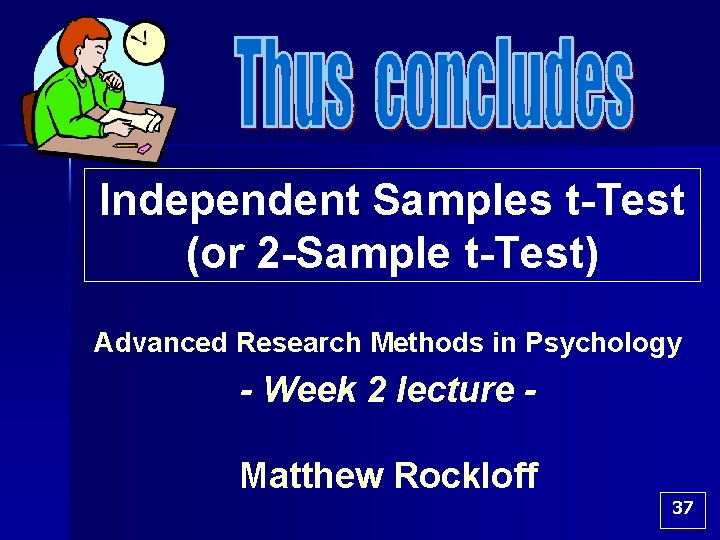Independent Samples t-Test (or 2 -Sample t-Test) Advanced Research Methods in Psychology - Week 2 lecture Matthew Rockloff 37